PHY.K02UF Molecular and Solid State Physics

## Fourier series in 3-D

There are fourteen Bravais lattices in three dimensions. A three dimensional periodic function can be described with a Fourier series of the form,

$$f(\vec{r})=\sum \limits_{\vec{G}} f_{\vec{G}}\exp \left(i\vec{G}\cdot\vec{r}\right),$$

where the Fourier coefficients are given by,

$$f_{\vec{G}} = \frac{1}{V_{\text{uc}}}\int\limits_{\text{unit cell}}f(\vec{r})\exp (-i\vec{G}\cdot \vec{r})d\vec{r}.$$

Example 1: cubes repeated on a bcc lattice

Cubes are arranged on a bcc lattice such that the corners of the cubes just touch. This is a three dimensional analog to the square wave problem that was solved in one dimension.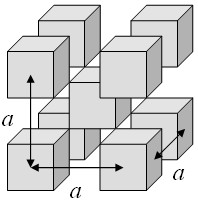A three-dimensional periodic function f is defined such that it has a constant value C inside the cubes and is zero outside the cubes. This function can be expressed as a Fourier series,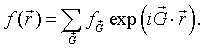The Fourier coefficients fG are given by,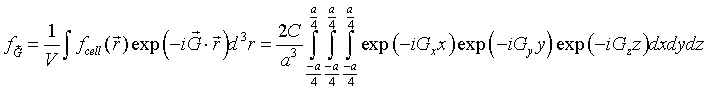The cube at the origin lies entirely within the Wigner-Seitz cell and the function f is zero outside the cube so we just need to integrate over the cube. The integrals over x, y and z are easily performed.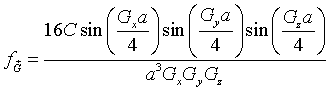This result can readily be generalized to any rectangular cuboid with dimensions Lx×Ly×Lz that is repeated on any three-dimensional Bravais lattice. As long as a primitive unit cell can be defined such that the cuboid is entirely within the cell, the Fourier series for a function that has the value C inside the cuboids and zero outside the cuboids is,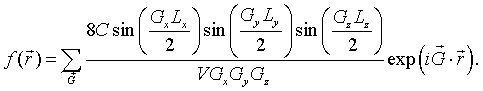Here V is the volume of the primitive unit cell.

Example 2: spheres on an fcc lattice

Spheres of radius R are arranged on a fcc lattice.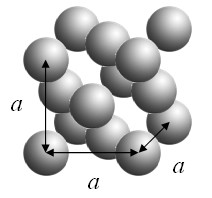A three-dimensional periodic function f is defined such that it has a constant value C inside the spheres and is zero outside the spheres. This function can be expressed as a Fourier series,The Fourier coefficients fG are given by,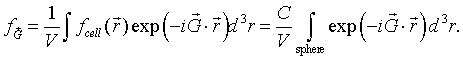As long as the spheres do not overlap, a primitive unit cell can be defined so that the sphere at the origin lies entirely within the primitive unit cell. Since the function f is zero outside sphere, we just need to integrate over the sphere.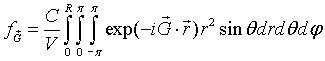The integral over φ is easily performed.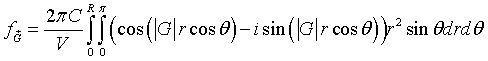Recognizing that,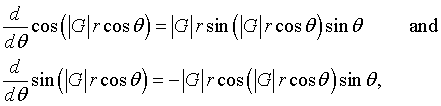the integral over θ can be performed.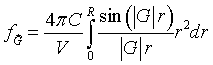Finally, performing the integral over r,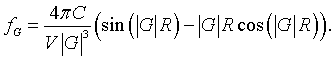As long as the spheres do not overlap, the Fourier series for spheres repeated on any Bravais lattice is,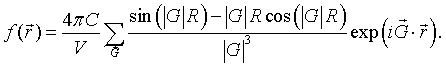Example 3: Total electron density

The total electron density in a crystal is a periodic function. Most of the electrons in a solid are core electrons that are very tightly bound to the nuclei. To a good approximation, the total electron density of an atom can be described by a Gaussian function $\frac{Z}{r_0^3 \pi^{3/2}}\exp (-r^2/r_0^2)$, where $Z$ is the number of electrons of the atom. The integral of this function over all space is $Z$. Typically the width $r_0$ of the Gaussian is so much smaller than the lattice constant $a$ the that the integral of this function over a unit cell is also about $Z$. The electron density of the basis of a crystal is,

$$n_{u.c.}(\vec{r})=\frac{1}{V_{\text{u.c.}}}\sum \limits_j \frac{Z_j}{r_{0j}^3 \pi^{3/2}}\exp \left(-\frac{(\vec{r}-\vec{r}_j)^2}{r_{0j}^2}\right),$$

where $j$ sums over the atoms in the basis and $\vec{r}_j$ is the position of atom $j$. The constant $\frac{Z_j}{r_{0j}^3 \pi^{3/2}}$ in front of the Gaussian is chosen so that when the electron density is integrated over the unit cell, the Gaussians around each atom contribute an amount $Z_j$ to the integral. The sum is then $\sum\limits_j Z_j$. Dividing this sum by the volume of the unit cell, $V_{u.c.}$, the electron density is obtained.

The Fourier coefficients can be found by multiplying both sides of this equation by $\exp (-i\vec{G}\cdot\vec{r})$ and integrating over a unit cell. The result is,

$\begin{equation} n_{\vec{G}}=\frac{1}{V_{\text{u.c.}}^2}\sum \limits_j Z_j\exp \left(-\frac{r_{0j}^2G^2}{4}\right)\exp (-i\vec{G}\cdot\vec{r}_j). \end{equation}$

The square of the Fourier coefficients $|n_{\vec{G}}|^2$ is proportional to the intensity of a diffraction peak in an x-ray diffraction experiment. Since the volume of the unit cell is the same for all of the Fourier coefficients and only their ratio is important for x-ray diffraction, it is usual to leave the factor of $1/V_{\text{u.c.}}^2$ out and define the structure factor $S_{\vec{G}}$ as,

$\begin{equation} S_{\vec{G}}= V_{\text{u.c.}}^2n_{\vec{G}}=\sum \limits_j Z_j\exp \left(-\frac{r_{0j}^2G^2}{4}\right)\exp (-i\vec{G}\cdot\vec{r}_j). \end{equation}$

The structure factor for $\vec{G}=0$ is the number of electrons per unit cell,

$\begin{equation} S_{\vec{G}=0}=\sum \limits_j Z_j. \end{equation}$

Since the Gaussians are sharply peaked around the nuclei, sometimes the electron density is approximated by collection of $\delta$-functions,

$\begin{equation} n_{u.c.}(\vec{r})=\frac{1}{V_{\text{u.c.}}}\sum \limits_j Z_j\delta(\vec{r}-\vec{r}_j). \end{equation}$

In this case, the structure factors are,

$\begin{equation} S_{\vec{G}}=\sum \limits_j Z_j\exp (-i\vec{G}\cdot\vec{r}_j). \end{equation}$

Example 4: The molecular orbital Hamiltonian

An important periodic function is the periodic potential that appears in the molecular orbital Hamiltonian for a crystal. For a crystal containing only one atom in the basis, the potential is,

$\begin{equation} U(\vec{r})=-\frac{Ze^2}{4\pi \epsilon_0}\sum \limits_j\frac{1}{|\vec{r}-\vec{r}_j|}. \end{equation}$

Here $\vec{r}_j$ are the positions of the atoms. The periodic potential is formed by the Coulomb potentials of the nuclei each with a positive charge of $Ze$. The Fourier series for this potential is,

$\begin{equation} U(\vec{r})=-\frac{Ze^2}{V_{u.c.} \epsilon_0}\sum \limits_{\vec{G}}\frac{\exp (i\vec{G}\cdot\vec{r})}{|\vec{G}|^2}. \end{equation}$

Example 5: Muffin tin potentials

In solid state physics, a 2-d muffin tin potential is a potential consisting of a lattice of circular regions. Within the circular regions the potential is a Coulomb potential with the form -Ze²/(4πεr) and between the circular regions the potential is constant. The constant is chosen so that there is no discontinuity in the potential. This potential looks like a muffin tin.

In three dimensions, a muffin tin potential U(r) is a lattice of spherical regions where the potential inside the the spheres is -Ze²/(4πεr) and outside the spheres the potential is constant.

The integral that must be performed to determine the Fourier coefficients is,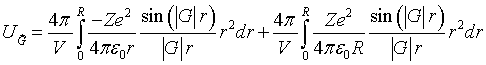The first term is the radial Fourier transform of the Coulomb potential and the second term adds a constant value to the potential in the spherical regions to match the Coulomb potential to the zero potential outside the spherical regions.

The Fourier series for a muffin tin potential is,

$$U(\vec{r})=\frac{Ze^2}{V\epsilon_0} \sum\limits_{\vec{G}}\left(\frac{\cos(|G|R)-1}{|G|^2}+\frac{\sin(|G|R)-|G|R\cos(|G|R)}{R|G|^3}\right)\exp\left(i\vec{G}\cdot\vec{r}\right).$$